Updating search results...

# 35 Results

View
Selected filters:
• Shodor Education Foundation## Algebra Four

Rating
0.0 stars
Overview:

In this activity, students practice solving algebraic equations.

Subject:
Mathematics
Material Type:
Interactive
Provider:
Shodor Education Foundation
Author:
Shodor
02/26/2019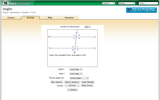Conditions of Use:

## Angles and Angle Vocabulary

Rating
0.0 stars
Overview:

In this interactive, students demonstrate their understanding of angle vocabulary.

Subject:
Mathematics
Material Type:
Interactive
Provider:
Shodor Education Foundation
03/24/2017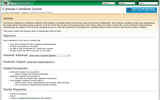## Cartesian Coordinate System

Rating
0.0 stars
Overview:

In this lesson, students will use the first quadrant of the coordinate ...

In this lesson, students will use the first quadrant of the coordinate plane to graph points.

Subject:
Mathematics
Material Type:
Lesson Plan
Provider:
Shodor Education Foundation
Author:
The Shodor Education Foundation, Inc
02/26/2019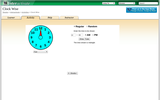## Clock Wise

Rating
1.0 stars
Overview:

For this interactive, students practice reading a clock, input times for the ...

For this interactive, students practice reading a clock, input times for the clock to display, or let the clock generate random times for them to read. Students choose from three difficulty levels.

Subject:
Mathematics
Material Type:
Interactive
Provider:
Shodor Education Foundation
Author:
Shodor Education Foundation
02/26/2019## Comparing Fractions

Rating
0.0 stars
Overview:

In this lesson, students learn how to compare fractions. Students begin by ...

In this lesson, students learn how to compare fractions. Students begin by drawing a picture to compare fractions then interact with a computer program.

Subject:
Mathematics
Material Type:
Lesson Plan
Provider:
Shodor Education Foundation
Author:
The Shodor Education Foundation, Inc
02/26/2019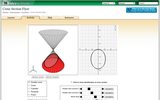Conditions of Use:

## Cross Section Flyer

Rating
0.0 stars
Overview:

Interactive applet that allows users to explore cross sections of different 3D ...

Interactive applet that allows users to explore cross sections of different 3D objects by manipulating the properties.

Subject:
Mathematics
Material Type:
Activity/Lab
Provider:
Shodor Education Foundation
02/27/2018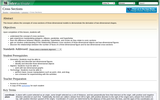## Cross Sections

Rating
0.0 stars
Overview:

This lesson utilizes the concepts of cross-sections of three-dimensional models to demonstrate ...

This lesson utilizes the concepts of cross-sections of three-dimensional models to demonstrate the derivation of two-dimensional shapes.

Subject:
Math 3
Material Type:
Activity/Lab
Lesson Plan
Provider:
Shodor Education Foundation
Author:
Shodor Education Foundation, Inc.
02/26/2019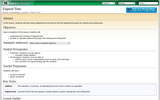## Elapsed Time

Rating
0.0 stars
Overview:

In this lesson, students will learn about elapsed time and how to ...

In this lesson, students will learn about elapsed time and how to find the elapsed time given the starting and ending time.

Subject:
Mathematics
Material Type:
Interactive
Lesson Plan
Provider:
Shodor Education Foundation
Author:
The Shodor Education Foundation
02/26/2019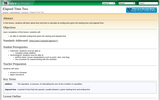## Elapsed Time Two

Rating
0.0 stars
Overview:

In this lesson, students explore elapsed time given a starting time and ...

In this lesson, students explore elapsed time given a starting time and the number of hours that passed.

Subject:
Mathematics
Material Type:
Activity/Lab
Provider:
Shodor Education Foundation
Author:
The Shodor Education Foundation, Inc
02/26/2019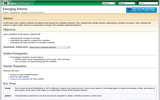## Emerging Patterns

Rating
0.0 stars
Overview:

In this lesson, students will generate and analyze an algebraic pattern. Students ...

In this lesson, students will generate and analyze an algebraic pattern. Students will describe two different patterns. The first pattern taken from Koch's Snowflakes and the second pattern taken from a population of wolves and rabbits.

Subject:
Mathematics
Material Type:
Lesson Plan
Provider:
Shodor Education Foundation
Author:
The Shodor Education Foundation, Inc
02/26/2019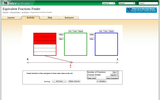## Equivalent Fractions Finder

Rating
0.0 stars
Overview:

In this interactive activity students are to visualize the concept of equivalent ...

In this interactive activity students are to visualize the concept of equivalent fractions. The student has to illustrate two fractions which have the same value as a third given fraction.

Subject:
Mathematics
Material Type:
Interactive
Provider:
Shodor Education Foundation
Author:
Shodor
02/26/2019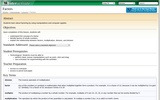## Factors

Rating
0.0 stars
Overview:

In this lesson, students will understand the concept of a factor. Students ...

In this lesson, students will understand the concept of a factor. Students will determine the factors that belong to a whole number.

Subject:
Mathematics
Material Type:
Lesson Plan
Provider:
Shodor Education Foundation
Author:
The Shodor Education Foundation, Inc
02/26/2019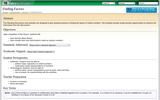## Finding Factors

Rating
0.0 stars
Overview:

In this lesson, students explore factors of whole numbers. Students work with ...

In this lesson, students explore factors of whole numbers. Students work with a computer program in order to practice factorization.

Subject:
Mathematics
Material Type:
Activity/Lab
Provider:
Shodor Education Foundation
Author:
The Shodor Education Foundation, Inc
02/26/2019## Finding Remainders with Pascal's Triangle

Rating
0.0 stars
Overview:

In this lesson, students explore remainders using Pascal's triangle.

Subject:
Mathematics
Material Type:
Lesson Plan
Provider:
Shodor Education Foundation
Author:
The Shodor Education Foundation, Inc
02/26/2019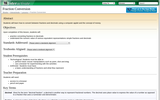## Fraction Conversion

Rating
0.0 stars
Overview:

In this lesson, students explore using decimals to represent fractional amounts. Students ...

In this lesson, students explore using decimals to represent fractional amounts. Students compare fractions to decimal amounts.

Subject:
Mathematics
Material Type:
Lesson Plan
Provider:
Shodor Education Foundation
Author:
The Shodor Education Foundation, Inc
02/26/2019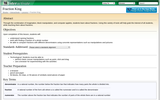## Fraction King

Rating
0.0 stars
Overview:

In this lesson, student explore fractions using themselves as manipulatives. Teacher reads ...

In this lesson, student explore fractions using themselves as manipulatives. Teacher reads the students a story about a King that divides his "subjects" into different groups creating fractions. Students discuss the fractional parts that are created and work in pairs to place small squares into equal groups.

Subject:
Mathematics
Material Type:
Lesson Plan
Provider:
Shodor Education Foundation
Author:
The Shodor Education Foundation, Inc
02/26/2019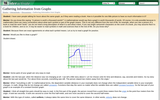## Gathering Information from Graphs

Rating
0.0 stars
Overview:

On this webpage, a simulated discussion about motion graphs between students and ...

On this webpage, a simulated discussion about motion graphs between students and a mentor takes place with sample graphs illustrated. The discussion explains how to read motion graphs and what information we can gather from them.

Subject:
Science
Material Type:
Lesson
Provider:
Shodor Education Foundation
Author:
The Shodor Education Foundation
02/26/2019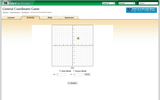## General Coordinates Game

Rating
2.0 stars
Overview:

This activity allows the user to plot points on the coordinate plane ...

This activity allows the user to plot points on the coordinate plane and to read the coordinates of a point plotted by the computer.

Subject:
Mathematics
Material Type:
Activity/Lab
Interactive
Provider:
Shodor Education Foundation
Author:
Shodor
02/26/2019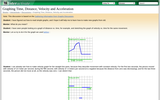## Graphing Time, Distance, Velocity, and Acceleration

Rating
0.0 stars
Overview:

On this webpage, a discussion takes place between students and a mentor ...

On this webpage, a discussion takes place between students and a mentor about making different types of motion graphs. Illustrations of the graphs discussed are included. This is the second in a series of discussions.

Subject:
Science
Material Type:
Lesson
Provider:
Shodor Education Foundation
Author:
The Shodor Education Foundation
02/26/2019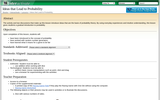## Ideas that Lead to Probability

Rating
0.0 stars
Overview:

The activity and two discussions that make up this lesson introduce ideas ...

The activity and two discussions that make up this lesson introduce ideas that are the basis of probability theory. By using everyday experiences and intuitive understanding, this lesson gives students a gradual introduction to probability.

Subject:
Mathematics
Material Type:
Activity/Lab
Lesson Plan
Provider:
Shodor Education Foundation
Author:
The Shodor Education Foundation